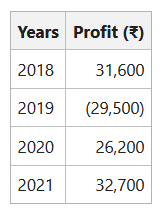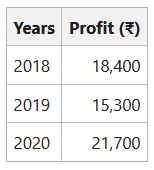Open in App
Not now

# Super Profit Method of Calculating Goodwill

• Last Updated : 18 Aug, 2022

Goodwill is the intangible asset of the business. An enterprise earns more profit than the normal profit because of Goodwill. Goodwill can be defined as the reputation of the business earned through hard work, honesty, quality, and customer satisfactory services. It helps to earn profits in the future without much effort because of the retention of the customers. Thus, when in the same industry, one firm earns more profit than the other firms, it is because of the existence of goodwill. It is an attractive force that brings old customers to that old place.

” Goodwill is the probability that the old customers will resort to the old place.”  – Lord Eldon

Meaning of Super Profit Method:

Super Profit is the excess of the average profit over the normal profit. Goodwill is calculated by multiplying the super profit with the agreed number of years’ purchase. Profit of the business yields from the capital employed. Some organisation earns more profit than other organisation from the capital investment in the organisation. Normal return is the percentage of capital employed of profit earned by a similar type of business. The average capital employed is taken into consideration to calculate super profit.

The Formula of Super Profit Method:

Super Profit = Actual Average Profit – Normal Profit

Goodwill = Super Profit x Number of Year Purchase

Steps of Super Profit Method of Calculating Goodwill:

1. Calculate the Average Capital Employed:

Average Capital Employed =Where, Capital Employed = Capital + Free Reserves – Fictitious Assets

2. Calculate the actually expected profit, i.e., average profit:

Average Expected Profit =3. Calculate the normal profit on average capital employed by:

Normal Profit =* Normal Rate of Return refers to the rate of return normally earned by other firms in the same industry.

4. Calculate the Super Profit:

Super Profit = Actual Average Profit – Normal Profit

5. Calculate the value of Goodwill:

Goodwill = Super Profit x Number of Years’ Purchase

Illustration 1:

The net profit of a firm earned during the past four years is as follows:The capital employed by the firm is ₹45,000 and the normal rate of return is 8%. Calculate the value of goodwill based on the past three years’ purchase of the average super profit for the last four years.

Solution

Calculating Average Expected Profit:

Average Expected Profit == ₹15,250

Calculating Normal Profit:

Normal Profit === ₹3,600

Calculating Super Profit:

Super Profit = Average Expected Profit – Normal Profit
= ₹15,250 – ₹3,600
= ₹11,650

Calculating Goodwill:

Goodwill = Super Profit x Number of years purchase
= ₹11,650 x 3
= ₹34,950

Illustration 2:

M and N are partners sharing profit and losses in the ratio of 2:3. P is the new partner to be admitted in the firm of 2/3rd share. For this purpose, goodwill has to be valued at two years’ purchase of super profit. The average maintainable profit of the firm is ₹53,000. The normal rate of return is to take 10% and the capital investment of the firm is valued at ₹1,94,600. Calculate the value of Goodwill.

Solution

Calculating Normal Profit:

Normal Profit == ₹19,460

Calculating Super Profit:

Super Profit = Average Expected Profit – Normal Profit
= ₹53,000 – ₹19,460
= ₹33,540

Calculating Goodwill:

Goodwill = Super Profit x Number of years purchase
= ₹33,540 x 2
= ₹67,080

Illustration 3:

A firm earned the net profits during the last three years as follows:The capital employed in the firm is ₹3,40,000, and a fair return on capital is 17%. Calculate the value of goodwill of the firm based on three years’ purchase of average super profit.

Solution:

Calculating Average Expected Profit:

Average Expected Profit === ₹21,800

Calculating Normal Profit:

Normal Profit === ₹17,000

Calculating Super Profit:

Super Profit = Average Expected Profit – Normal Profit
= ₹21,800 – ₹17,000
= ₹4,800

Calculating Goodwill:

Goodwill = Super Profit x Number of years purchase
= ₹4,800 x 3
= ₹14,400

My Personal Notes arrow_drop_up
Related Articles